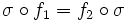# Function property

This article is about a general term. A list of important particular cases (instances) is available at Category:Function properties

BEWARE! This term is nonstandard and is being used locally within the wiki. [SHOW MORE]

## Definition

A function property is a map from the collection of all possible functions from a group to itself, to the two-element set (true, false). A function which gets mapped to true is said to have the function property, and a function which gets mapped to false is said to not have the function property.

The function property must satisfy isomorphism-invariance: if$f_1:G \to G$ and$f_2:H \to H$ are functions, and there is an isomorphism$\sigma:G \to H$ such that$\sigma \circ f_1 = f_2 \circ \sigma$, then$f_1$ satisfies the function property iff$f_2$ satisfies the function property.